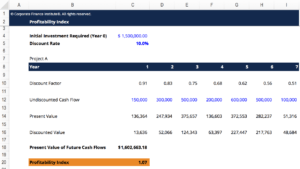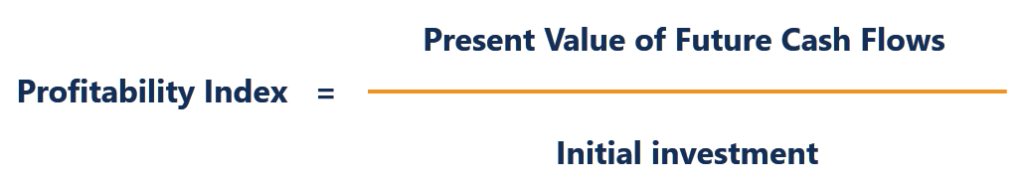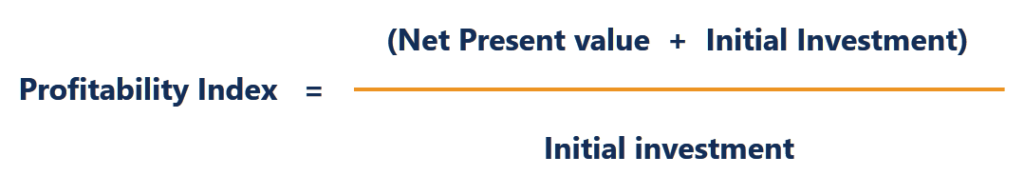# Profitability Index Template

The ratio between the present value of future cash flows to the initial investment

## Profitability Index Template

This Profitability Index (PI) template will help visualize the Present Value of future cash flows, which will then be used to calculate the PI of the project. The PI measures the ratio between the present value of future cash flows to the initial investment. Other names for Profitability Ratio are Profit Investment Ratio (PIR) and Value Investment Ratio (VIR).

Here is a picture of the template:### Profitability Index Formula

The formula for the PI is as follows:orTherefore:

• If the PI is greater than 1, the project generates value and the company should proceed with the project.
• If the PI is less than 1, the project destroys value and the company should not proceed with the project.
• If the PI is equal to 1, the project breaks even and the company is indifferent between proceeding and not proceeding with the project.

The higher the profitability index, the more attractive the investment.

### More Free Templates

For more resources, check out our business templates library to download numerous free Excel modeling, PowerPoint presentation, and Word document templates.

• Excel Modeling Templates
• PowerPoint Presentation Templates
• Transaction Document Templates